# Dividing Mixed Numbers

Example 1: Jack has a piece of wood that is 12 and 2/3 inches long. He divides the wood into pieces that are 3 and 4/5 inches long. How many pieces will he have?

Analysis: We need to divide these mixed numbers in order to solve this problem.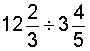Solution: First we will convert each mixed number to an improper fraction. Then we can divide those fractions.Step 1: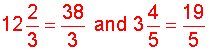Step 2: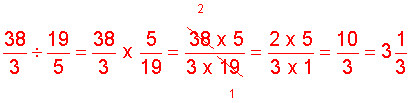Answer: Jack will have 3 and 1/3 pieces.

Example 2: Divide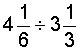Analysis: First convert each mixed number to an improper fraction. Then divide.

Step 1: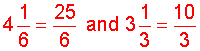Step 2: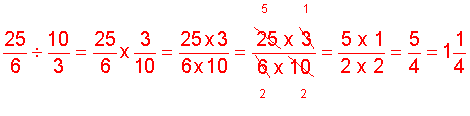The following is the procedure for dividing mixed numbers.

Procedure: To divide mixed numbers, first convert each mixed number to an improper fraction, then divide the first fraction by the second. Simplify your result, if necessary.

Let's look at some examples using this procedure.

Example 3: Divide.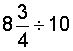Step 1: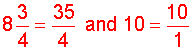Step 2: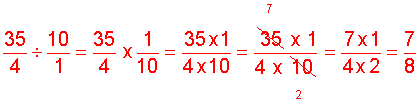Example 4: Divide.Step 1: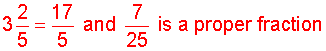Step 2: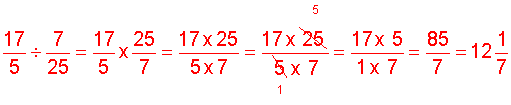Example 5: Divide.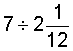Solution: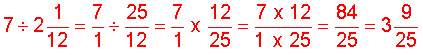Summary: To divide mixed numbers, first convert each mixed number to an improper fraction, then divide the first fraction by the second. Simplify your result, if necessary.

### Exercises

Directions: In each exercise below, divide the mixed numbers. Be sure to simplify your result, if necessary. Click once in an ANSWER BOX and type in your answer; then click ENTER. After you click ENTER, a message will appear in the RESULTS BOX to indicate whether your answer is correct or incorrect. To start over, click CLEAR.

Note: To write the mixed number four and two-thirds, enter 4, a space, and then 2/3 into the form.

 1.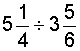ANSWER BOX:   RESULTS BOX:
 2.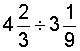ANSWER BOX:   RESULTS BOX:
 3.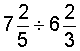ANSWER BOX:   RESULTS BOX:
 4.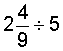ANSWER BOX:   RESULTS BOX:
 5. A large pitcher holds 15 liters of water. If each glass holds 2 and 1/2 liters, then how many glasses of water can be poured from the pitcher? ANSWER BOX:   glasses  RESULTS BOX:
 Lessons on Multiplying and Dividing Fractions and Mixed Numbers 1. Multiplying Fractions 2. Multiplying Fractions by Cancelling Common Factors 3. Multiplying Mixed Numbers 4. Reciprocals 5. Dividing Fractions 6. Dividing Mixed Numbers 6. Solving Word Problems 7. Practice Exercises 8. Challenge Exercises 9. Solutions